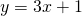# Arithmetic Sequences: Two Terms

You may have noticed that arithmetic sequences have much in common with straight line graphs.

Compare the sequencewith the straight line.

What kind of value doesrepresent in the sequence? What kind of value doesrepresent in the linear equation?

If you are given two points on a straight line, or if you are given two terms in an arithmetic sequence, you can calculate the equation.

The best algebraic technique for you (the one that makes most sense initially) is often the one that you arrive at yourself. Try the applet, and describe any method you come up with!

I’m done here! Take me back to the Sequences and Series Menu!